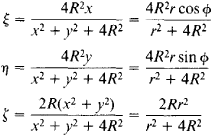# Riemann Sphere

(redirected from Extended complex plane)

## Riemann sphere

[′rē‚män ‚sfir]
(mathematics)
The two-sphere whose points are identified with all complex numbers by a stereographic projection. Also known as complex sphere.
McGraw-Hill Dictionary of Scientific & Technical Terms, 6E, Copyright © 2003 by The McGraw-Hill Companies, Inc.
The following article is from The Great Soviet Encyclopedia (1979). It might be outdated or ideologically biased.

## Riemann Sphere

a geometric representation introduced by B. Riemann for the set of complex numbers. The complex number

z = x + iy = r(cos ɸ + i sin ɸ) = re

can be represented by the point in the complex plane with the Cartesian coordinates x,y or the polar coordinates r, ɸ. To construct a Riemann sphere, a sphere is placed so as to be tangent to the complex plane at the origin of coordinates, and the points of the plane are mapped onto the surface of the sphere by means of a stereographic projection.

Each complex number is thus represented by a corresponding point of the sphere, which is called a Riemann sphere. The number 0 here is represented by the south pole of the Riemann sphere. Numbers with the same argument ɸ = constant (rays in the complex plane) are represented on the sphere by meridians. Numbers with the same modulus r = constant (circles in the complex plane) are represented by parallels of the Riemann sphere. No point of the complex plane corresponds to the north pole of the Riemann sphere. To preserve the one-to-one correspondence between points of the complex plane and the Riemann sphere, there is adjoined to the plane a “point at infinity,” which is assumed to correspond to the north pole and designated by z = ∞. Thus, the complex plane contains a single point at infinity, in contrast to a projective plane.

Suppose a system of rectangular Cartesian coordinates ξ, n, ζ is introduced in such a way that the ξ-axis and η-axis coincide with the x-axis and y-axis, respectively. There then corresponds to the point x + iy of the complex plane the pointof the Riemann sphere. The equation of the sphere is ξ2 + η2 + ζ2 - 2Rζ = 0.

The Great Soviet Encyclopedia, 3rd Edition (1970-1979). © 2010 The Gale Group, Inc. All rights reserved.
Mentioned in ?
References in periodicals archive ?
Value distribution of L-functions concerns distribution of zeros of L-functions L and, more generally, the c-points of L, i.e., the roots of the equation L(s) = c, or the points in the pre-image [L.sup.-1] = {s [member of] C : L(s) = c}, where and in what follows, s denotes a complex variable in the complex plane C and c denotes a value in the extended complex plane C [union] {[infinity]}.
Let a be an any value in the extended complex plane and let k be an arbitrary nonnegative integer.
We study conformal maps from multiply connected domains in the extended complex plane onto lemniscatic domains.
Let K be any simply connected domain (open and connected set) in the extended complex plane [??] with [infinity] [member of] K and with at least two boundary points.
Suppose that u is a nontrivial solution of equation (6) and that f is the Newton's method of u, then for any [alpha] [member of] [??], where [??] is the extended complex plane, we have [delta]([alpha], f) = 0.
We call W a hyperbolic domain if [??]\W contains at least three points, where [??] is the extended complex plane. For an a [member of] C\W, define [C.sub.W] (a) = inf{[[lambda].sub.W] (z)[absolute value of (z - a)]: [for all]z [member of] W}, where [[lambda].sub.W] (z) is the hyperbolic density on W.

Site: Follow: Share:
Open / Close